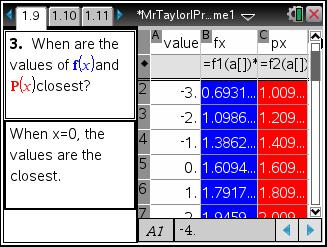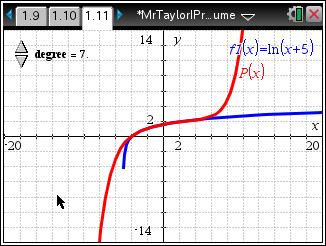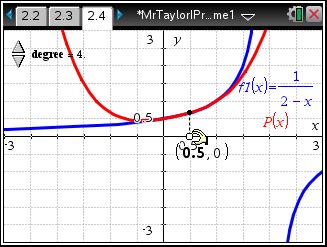# Activities

••• ##### Subject Area

• Math: Calculus: Series and Taylor Polynomials

• ##### Author9-12

45 Minutes

• ##### Device
• TI-Nspire™ Navigator™
• TI-Nspire™ CAS
•TI-Nspire™ CX/CX II
•TI-Nspire™ CX CAS/CX II CAS
• ##### Software

TI-Nspire™ CAS

3.2

By Pat Flynn

## Mr. Taylor, I Presume?

#### Activity Overview

Students will explore Taylor polynomials graphically and analytically, as well as graphically determine the interval where the Taylor polynomial approximates the function it models.

#### Key Steps

•In this problem, students investigate a Taylor polynomial centered at zero. Students will recall that this Taylor polynomial is also known as a Maclaurin polynomial.

Students find the 4th degree Taylor polynomial that approximates f(x) = ln(x + 5) at x = 0. They will notice that the values are closest at the center and become farther apart the further the x-values are from the center.

•This problem also gives students the opportunity to explore different degrees of a Taylor polynomial. They use a slider to change the degree of the polynomial, which changes the graph.

This will demonstrate that a Taylor polynomial will only approximate the function over a given interval, no matter how large the Taylor Polynomial is.

•In Problem 2, students work a problem where the Taylor polynomial is not centered at zero. They see that the polynomial follows the original function for a much smaller interval and they should use (xa)n instead of xn.

At the end of this activity, students will be able to find the first few terms of a Taylor series approximation to a function for any given value of x. They will also be able to graph a function and its Taylor polynomials of various degrees to show their convergence to a function.